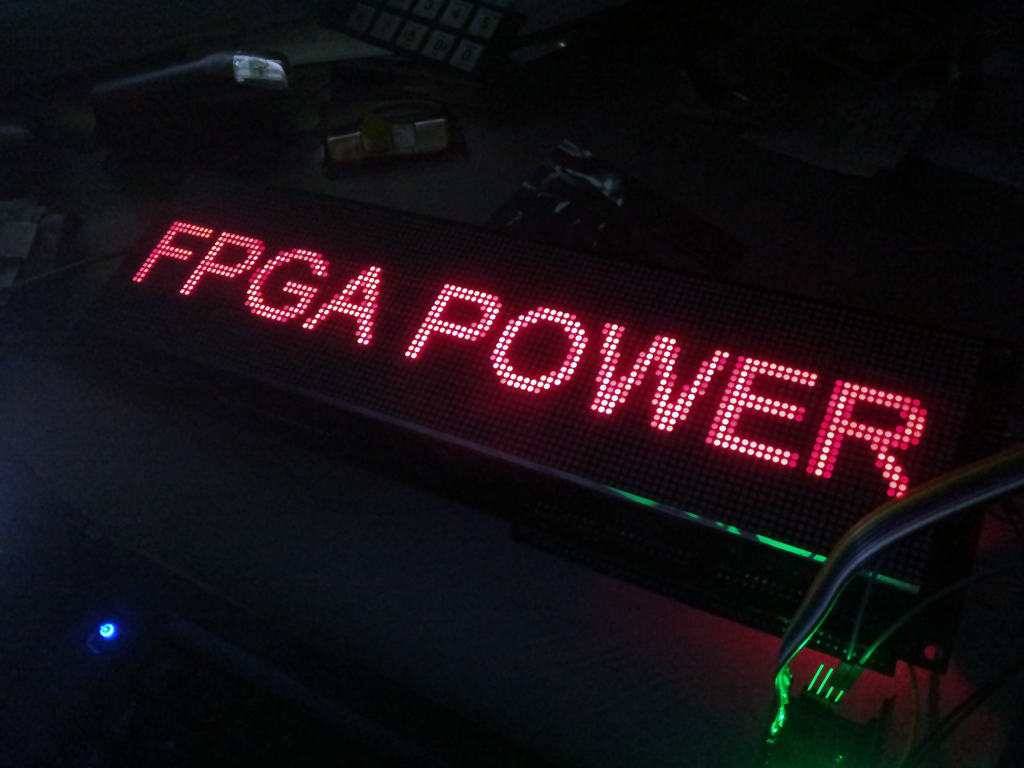# LEDDMD 128×32 Complete

So I finally finished the prototype of the 128 x 32 Light Emitting Diode Dot Matrix Display V1.0 (LEDDMD). The protocol to write to the display is almost exactly like a shift register. There is a clock, latch, and data lines. It works in either 1 bit mode or 8 bit mode data line modes.

In 1 bit mode there is only 1 data line and in 8 bit mode this is 8 data lines. 8 bit mode enables you to clock in an entire byte of data at a time speeding up the transfer process by a factor of 8. In either mode you can do animations smoothly. All processing of the data is done on the microcontroller. The Display stores the data and takes care of running the display.

The bulk of the hardware is in the FPGA. I am using a Cyclone II EP2C8Q208C8N FPGA breakout board. There are some darlington transistor arrays that sink the current from a single row. To expand the I/O of the FPGA some decoders are used.

Here are the links to all the code:
FPGA:
Main Routine
Memory

Propeller:
Transmission Protocol and Test# 128×32 DMD Update

The verilog code is almost fully debugged. The demo code that will run on a Parallax Propeller is in the works. Right now the demo is fairly basic. Today I wrote a C program that takes a 4-bit bitmap image and strips out the header and and converts it to a 2-bit image. It then reorientates the data so the image is “correct”. Bitmap images data reads the image from bottom left to top right. This is essentially backwards. So the program corrects this which means less work for the microcontroller and faster transmission of pixels.

# Updated Code for the 128×32 DMD

The old program took to make Logic elements to fit. Now it uses a 2-port RAM element to replace the huge registers. The protocol for shifting in is a bit different now. Instead of shifting in all the data at once and then Latching the user shifts in the data 64 bits at a time then latches.

``````
module LED_Matrix_32x128
(
clk,e_cnt,row_cnt,col,SDRAM_CS,SRAM_CS,LAT,INPUT,SCLK
);

input  wire  clk;
input  wire  LAT;
input  wire  [7:0]  INPUT;
input  wire  SCLK;

output  reg [63:0]  col;
output  reg [0:0]   SDRAM_CS;
output  reg [0:0]   SRAM_CS;
output  reg [5:0]  row_cnt;
output  reg [4:0]  e_cnt;

reg [24:0]  clk_slow;
reg [63:0]  col_buffer;

reg  [63:0]  disp_mem_data;
reg      disp_mem_wren;
wire  [63:0]  disp_mem_q;
initial
begin
SDRAM_CS <= 1'b0;
SRAM_CS <= 1'b0;
clk_slow <= 16'b0000000000000000;
row_cnt <= 5'b00000;
e_cnt <= 4'b1000;
disp_mem_wren <= 0;
end

always @(posedge clk)
begin
clk_slow <= clk_slow + 1'b1;
end

always @(posedge clk_slow)
begin
if(row_cnt > 5'b01111)
begin
row_cnt <= 5'b00000;
e_cnt <= {e_cnt[2:0],e_cnt};
end

col <= disp_mem_q;
row_cnt <= row_cnt + 1'b1;

end

always @(posedge SCLK)
begin
if(LAT)
begin
col_buffer <= {col_buffer[62:0],INPUT};
end
else
begin
disp_mem_data <= col_buffer;
disp_mem_wren <= 1;
disp_mem_wren <= 0;
end
end

display_memory disp_mem_inst (
.clock  (clk),
.data   (disp_mem_data),
.wren   (disp_mem_wren),
.q      (disp_mem_q)
);

endmodule
``````

# 128×32 DMD Verilog Code

Here is some Verilog code for a 32×128 DMD I am designing.

``````
module LED_Matrix_32x128
(
clk,e_cnt,row_cnt,col,SDRAM_CS,SRAM_CS,LAT,DATA,SCLK
);

input  wire    clk;
input  wire    LAT;
input  wire    DATA;
input  wire    SCLK;

output  reg [63:0]  col;
output  reg [0:0]   SDRAM_CS;
output  reg [0:0]   SRAM_CS;
output  reg [5:0]  row_cnt;
output  reg [4:0]  e_cnt;

reg [24:0]  clk_slow;
reg [4096:0]  col_buffer;
reg [63:0]  col_temp [63:0];

initial
begin
col <= 16'b0000000000000000;
SDRAM_CS <= 1'b0;
SRAM_CS <= 1'b0;
clk_slow <= 16'b0000000000000000;
row_cnt <= 5'b00000;
e_cnt <= 4'b1000;
end

always @(posedge clk) clk_slow=clk_slow + 1'b1;

always @(posedge clk_slow)
begin
if(row_cnt > 5'b01111)
begin
row_cnt <= 5'b00000;
e_cnt <= {row[2:0],row};
end
col <= col_temp[row_cnt];
row_cnt <= row_cnt + 1'b1;
end

always @(posedge SCLK)
begin
if(LAT)
begin
col_buffer <= {col_buffer[4094:0],DATA};
end
else
begin
col_temp  <= col_buffer [4095:4032];
col_temp  <= col_buffer [4031:3968];
col_temp  <= col_buffer [3967:3904];
col_temp  <= col_buffer [3903:3840];
col_temp  <= col_buffer [3839:3776];
col_temp  <= col_buffer [3775:3712];
col_temp  <= col_buffer [3711:3648];
col_temp  <= col_buffer [3647:3584];
col_temp  <= col_buffer [3583:3520];
col_temp  <= col_buffer [3519:3456];
col_temp  <= col_buffer [3455:3392];
col_temp  <= col_buffer [3391:3328];
col_temp  <= col_buffer [3327:3264];
col_temp  <= col_buffer [3263:3200];
col_temp  <= col_buffer [3199:3136];
col_temp  <= col_buffer [3135:3072];
col_temp  <= col_buffer [3071:3008];
col_temp  <= col_buffer [3007:2944];
col_temp  <= col_buffer [2943:2880];
col_temp  <= col_buffer [2879:2816];
col_temp  <= col_buffer [2815:2752];
col_temp  <= col_buffer [2751:2688];
col_temp  <= col_buffer [2687:2624];
col_temp  <= col_buffer [2623:2560];
col_temp  <= col_buffer [2559:2496];
col_temp  <= col_buffer [2495:2432];
col_temp  <= col_buffer [2431:2368];
col_temp  <= col_buffer [2367:2304];
col_temp  <= col_buffer [2303:2240];
col_temp  <= col_buffer [2239:2176];
col_temp  <= col_buffer [2175:2112];
col_temp  <= col_buffer [2111:2048];
col_temp  <= col_buffer [2047:1984];
col_temp  <= col_buffer [1983:1920];
col_temp  <= col_buffer [1919:1856];
col_temp  <= col_buffer [1855:1792];
col_temp  <= col_buffer [1791:1728];
col_temp  <= col_buffer [1727:1664];
col_temp  <= col_buffer [1663:1600];
col_temp  <= col_buffer [1599:1536];
col_temp  <= col_buffer [1535:1472];
col_temp  <= col_buffer [1471:1408];
col_temp  <= col_buffer [1407:1344];
col_temp  <= col_buffer [1343:1280];
col_temp  <= col_buffer [1279:1216];
col_temp  <= col_buffer [1215:1152];
col_temp  <= col_buffer [1151:1088];
col_temp  <= col_buffer [1087:1024];
col_temp  <= col_buffer [1023:960];
col_temp  <= col_buffer [959:896];
col_temp  <= col_buffer [895:832];
col_temp  <= col_buffer [831:768];
col_temp  <= col_buffer [767:704];
col_temp  <= col_buffer [703:640];
col_temp  <= col_buffer [639:576];
col_temp  <= col_buffer [575:512];
col_temp  <= col_buffer [511:448];
col_temp  <= col_buffer [447:384];
col_temp  <= col_buffer [383:320];
col_temp  <= col_buffer [319:256];
col_temp  <= col_buffer [255:192];
col_temp  <= col_buffer [191:128];
col_temp  <= col_buffer [127:64];
col_temp  <= col_buffer [63:0];
end
end
endmodule
``````